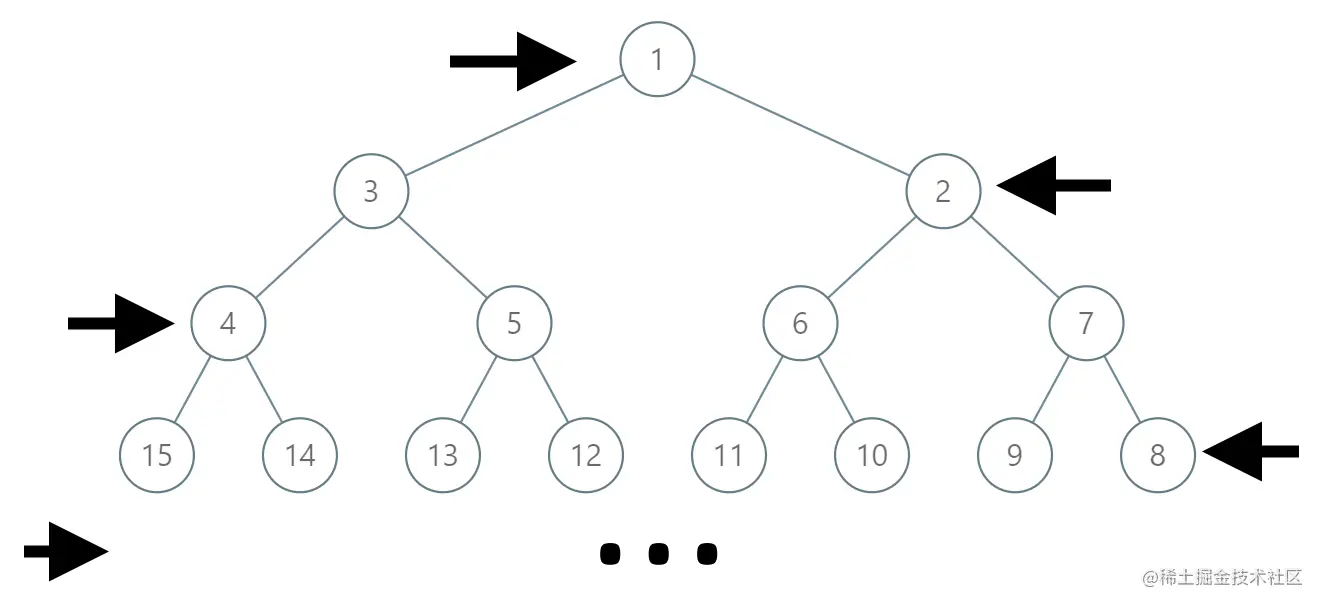# leetcode 1104. Path In Zigzag Labelled Binary Tree（python）

2022-01-30 05:29:38

This article has participated in 「 Digging force Star Program 」, Win a creative gift bag , Challenge creation incentive fund .

### describe

In an infinite binary tree where every node has two children, the nodes are labelled in row order.

In the odd numbered rows (ie., the first, third, fifth,...), the labelling is left to right, while in the even numbered rows (second, fourth, sixth,...), the labelling is right to left.Given the label of a node in this tree, return the labels in the path from the root of the tree to the node with that label.

Example 1:

``````Input: label = 14
Output: [1,3,4,14]
Copy code ``````

Example 2:

``````Input: label = 26
Output: [1,2,6,10,26]
Copy code ``````

Note:

``````1 <= label <= 10^6
Copy code ``````

### analysis

According to the meaning , An infinite full binary tree is given , All nodes are from 1 The starting integer is labeled , And in the odd rows of the tree, it is normal to arrange numbers from left to right , But in the even rows of the tree, the numbers are arranged from right to left , Now the title gives a target number label , Let's find the root node in this tree root To label List of nodes .

In fact, this problem is a problem of finding rules , Just set it on the tree structure . If the tree in the question is a tree with normal node numbers from left to right , Then you can see that you know the target node label after , Push back its parent nodes are label// 2 , All the way to the root node 1 until , If the goal label by 7 , The result is [1,3,7] , The tree structure is as follows ：

``````	1
2	    3
4   5   6   7

Copy code ``````

But now the trees are arranged inversely on even rows , The tree structure is as follows ：

``````	1
3	    2
4   5   6   7
Copy code ``````

So we just need to know from label To root On the passing node , What is the number in the original position can solve this problem , By looking for rules, we find that the target is on even or odd lines , A label label The number in the original position of is

``````2 ** (level) - 1 + 2 ** (level-1) - label
Copy code ``````

level Is the depth of the current node ,label Is the value of the current node , The calculated value is the original value of the current position , Remove it 2 Take an integer to know the value of its parent node . Keep repeating this to find the original value of the current position 、 And besides 2 Take an integer to get the operation of the parent node , Until the number of layers reaches the first layer , Then put all the nodes found in ascending order into the list result You can return in .

``````class Solution(object):
def pathInZigZagTree(self, label):
"""
:type label: int
:rtype: List[int]
"""
result = [label]
level = int(math.log(label, 2)) + 1
while level>1:
origin = 2 ** (level) - 1 + 2 ** (level-1) - label
label = origin // 2
result.append(label)
level -= 1
result = result[::-1]
return result

Copy code ``````

### Running results

``````Runtime: 12 ms, faster than 92.11% of Python online submissions for Path In Zigzag Labelled Binary Tree.
Memory Usage: 13.4 MB, less than 63.16% of Python online submissions for Path In Zigzag Labelled Binary Tree.
Copy code ``````

### analysis

This method is a way to see in the Forum , Our result can actually be seen as from label To the root node root The process of , As the goal in the question label by 14 It can be regarded as binary 1110 , Its parent node on the tree is 4（100） ,4 The parent node of is 3（11）,3 The parent node of is 1（1） It can be found that there is a relationship between the binary value of the parent node and the binary value of the current node ：

``````parent = 1 + inverted(label[1:-1])
Copy code ``````

inverted For the function , take 1 Turn into 0 , take 0 Turn into 1 . Keep repeating this calculation process , According to this law, we can solve the problem .

``````class Solution(object):
def pathInZigZagTree(self, label):
"""
:type label: int
:rtype: List[int]
"""
res = []
while label != 1:
res.append(label)
label = int('1' + "".join(map(lambda x: '1' if x == '0' else '0', format(label, 'b')[1:-1])), 2)
res.append(1)
return reversed(res)
Copy code ``````

### Running results

``````Runtime: 37 ms, faster than 21.05% of Python online submissions for Path In Zigzag Labelled Binary Tree.
Memory Usage: 13.3 MB, less than 63.16% of Python online submissions for Path In Zigzag Labelled Binary Tree.
Copy code ``````